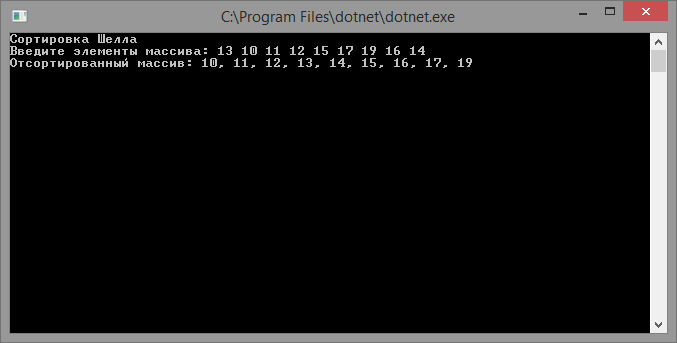# Shell sort

Updated: 15.09.2019

Shell sort – an array sorting algorithm that generalizes sorting by inserts.

## Algorithm Description

Shell’s sorting algorithm is based on two ideas:

• Insertion sorting shows the best results on practically ordered data arrays;
• Insertion sorting is ineffective for mixed data, because in one iteration the elements are shifted only one position.

To eliminate the shortcomings of the Insertion Sort algorithm, several sortings by inserts are performed in Shell sorting. Moreover, in each iteration, elements are compared that are located at different distances from each other, starting from the most distant (d = 12 of the array length) to comparing neighboring elements (d = 1).

## Shell sort implementation

``````using System;

class program
{
// method for exchanging elements
static void Swap(ref int a, ref int b)
{
var t = a;
a = b;
b = t;
}

static int[] ShellSort(int[] array)
{
// distance between elements that are compared
var d = array.Length / 2;
while (d >= 1)
{
for (var i = d; i < array.Length; i++)
{
var j = i;
while ((j >= d) && (array[j - d] > array[j]))
{
Swap(ref array[j], ref array[j - d]);
j = j - d;
}
}

d = d / 2;
}

return array;
}

static void Main(string[] args)
{
Console.WriteLine("Shell Sort");
Console.Write("Enter elements of the array:");
var s = Console.ReadLine().Split(new[] { "", ",", ";" }, StringSplitOptions.RemoveEmptyEntries);
var array = new int[s.Length];
for (int i = 0; i < s.Length; i++)
{
array[i] = Convert.ToInt32(s[i]);
}

Console.WriteLine("Sorted array: {0}", string.Join(",", ShellSort(array)));

The result of the program: